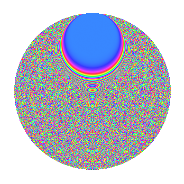# Properties

 Label 2100.2.doLevel 2100 Weight 2 Character orbit do Rep. character $$\chi_{2100}(47,\cdot)$$ Character field $$\Q(\zeta_{60})$$ Dimension 7552 Sturm bound 960

# Related objects

## Defining parameters

 Level: $$N$$ $$=$$ $$2100 = 2^{2} \cdot 3 \cdot 5^{2} \cdot 7$$ Weight: $$k$$ $$=$$ $$2$$ Character orbit: $$[\chi]$$ $$=$$ 2100.do (of order $$60$$ and degree $$16$$) Character conductor: $$\operatorname{cond}(\chi)$$ $$=$$ $$2100$$ Character field: $$\Q(\zeta_{60})$$ Sturm bound: $$960$$

## Dimensions

The following table gives the dimensions of various subspaces of $$M_{2}(2100, [\chi])$$.

Total New Old
Modular forms 7808 7808 0
Cusp forms 7552 7552 0
Eisenstein series 256 256 0

## Trace form

 $$7552q - 20q^{4} - 20q^{9} + O(q^{10})$$ $$7552q - 20q^{4} - 20q^{9} - 48q^{10} - 24q^{12} - 12q^{16} - 16q^{18} - 24q^{21} - 80q^{22} - 32q^{25} - 16q^{28} + 22q^{30} - 48q^{33} + 8q^{36} - 32q^{37} + 12q^{40} + 6q^{42} - 48q^{45} - 12q^{46} - 30q^{54} - 8q^{57} + 32q^{58} + 80q^{60} - 72q^{61} - 80q^{64} - 90q^{66} + 64q^{70} + 28q^{72} - 96q^{73} - 44q^{78} - 12q^{81} + 36q^{82} - 20q^{84} - 128q^{85} + 112q^{88} - 40q^{93} - 60q^{94} - 18q^{96} + O(q^{100})$$

## Decomposition of $$S_{2}^{\mathrm{new}}(2100, [\chi])$$ into newform subspaces

The newforms in this space have not yet been added to the LMFDB.

## Hecke characteristic polynomials

There are no characteristic polynomials of Hecke operators in the database